Courses

# Order and Molecularity of a Reaction Class 12 Notes | EduRev

## Class 12: Order and Molecularity of a Reaction Class 12 Notes | EduRev

The document Order and Molecularity of a Reaction Class 12 Notes | EduRev is a part of the Class 12 Course Chemistry Class 12.
All you need of Class 12 at this link: Class 12

Order of a Reaction

By performing a reaction in actual in laboratory and carefully examining it, it is possible to express the rate law as the product of concentrations of reactants each raised to some power.
For example consider the reaction : aA+ bB → cC +dD. The differential rate law is written as :

Rate =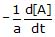=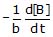=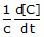=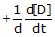= kr[A]m[B]n

where kr is called as rate constant of the reaction or velocity constant or specific Reaction rate.

k is a characteristic of a reaction at a given temperature. It changing only when the temperature changes.

The powers m and are integers or fractions. is called as order of reaction with respect to A and n is called as order of reaction with respect to B.The overall order of reaction = m n

Hence, the sum of powers of the concentration of the reactants in the rate law expression is called the order of that chemical reaction.

•  The values of m and n are calculated from the experimental data obtained for a reaction and the powers m and n are not related to the stoichiometric coefficients of the reactants
•  Order can be fractional, zero or negative.

For example consider the following reaction :

(i) H2(g) Br2(g) → 2 HBr (g) rate = k[H2] [Br2]1/2 (by experiment), order of reaction = 1 1/2 = 3/2

(ii)  CH3CHO(g) → CH4(g) CO(g), rate = k[CH3CHO]3/2 , order of reaction = 3/2

Units of k:

In general, the rate law for a nth order reaction can be taken as :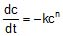where k : rate constant; c : concentration and n : order of reaction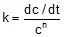⇒ Units of k º (mol/L)1-n (time)-1

For a 'zero' order reaction (n = 0) : Units of k = (mol/L)1 (time)-1 or mol/L/sec

For a first order reaction (n = 1) : Units of k º (time)-1 e.g. sec-1, min-1, hrs-1 etc.

For a second order reaction (n = 2) : Units of k º (mol/L)-1 (time)-1 or L/mol/sec.

Molecularity

As already discussed, the order of a reaction is an experimental concept.

A complex chemical reaction is understood in terms of various indirect steps called elementary processes. The study of a reaction in terms of elementary processes is called as reaction mechanism. Now various elementary steps occur at different rates.

The number of reacting species (atoms, ions or molecules) taking part in an elementary reaction, which must collide simultaneously in order to bring about a chemical reaction is called molecularity of a reaction.

In the rate determining step, when one molecule takes part, it is said to be a unimolecular reaction ; two molecules take part, it is said to be a bimolecular reaction; three molecules take part, it is said to be a termolecular reaction.

Unimolecular

1. Cyclopropane → propene

2. O3(g) → O2(g) + O(g)

3. N2O5(g) → N2O4(g) +1/2O2(g)

Bimolecular

1. NO(g) + O3 (g) → NO2(g)+ O2(g)

2. 2HI(g) → H2(g) I2(g)

Termolecular

1. 2NO(g) + O2(g) → 2NO2 (g)

The probability that more than three molecules can collide and react simultaneously is very small. Hence, reactions with the molecularity three are very rare and slow to proceed. It is, therefore, evident that complex reactions involving more than three molecules in the stoichiometric equation must take place in more than one step.

KClO3 + 6FeSO4 +3H2SO4 → KCl +3Fe2(SO4)3 + 3H2O

This reaction which apparently seems to be of tenth order is actually a second order reaction. This shows that this reaction takes place in several steps. Which step controls the rate of the overall reaction? The question can be answered if we go through the mechanism of reaction, for example, chances to win the relay race competition by a team depend upon the slowest person in the team. Similarly, the overall rate of the reaction is controlled by the slowest step in a reaction called the rate determining step.

(i) Order of a reaction is an experimental quantity. It can be zero and even a fraction but molecularity cannot be zero or a non integer.

(ii) Order is applicable to elementary as well as complex reactions whereas molecularity is applicable only for elementary reactions. For complex reaction molecularity has no meaning. 103 Chemical Kinetics

(iii) For complex reaction, order is given by the slowest step and molecularity of the slowest step is same as the order of the overall reaction.

•  For a reaction : A → B in the rate law : rate = k[A]m [B]n

Neither the order of reaction (m n) nor the molecularity of a reaction can be predicted from stoichiometric coefficient of a balanced reaction. The order of reaction is always to be determined experimentally and molecularity is determined theoretically after studying the reaction mechanism. However as a theoretical idea sometime, we can have an approximate order of reaction equal to molecularity (i.e., the number of molecules taking part in slowest elementary for complex reactions).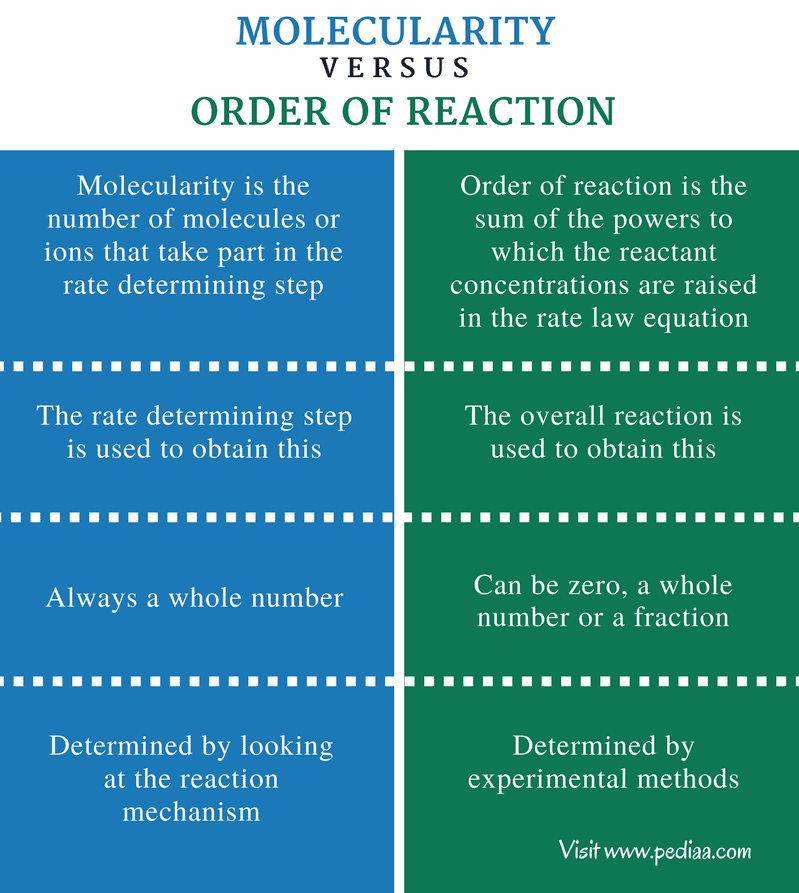Problem 1 :

The rate of formation of NO(g) in the reaction NOBr(g)→ NO(g) Br2(g) is found to be 1.6 × 10-4 M/s. Find the rate of overall reaction rate and rate of consumption of NOBr.

We have :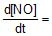1.6 × 10-4 M/s.

First write a balanced chemical equation. 2NOBr(g) → 2NO(g) Br2(g)

Now, Rate of overall reaction =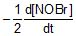=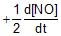=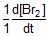= 0.8 × 10-4 M/s

Rate of consumption of NOBr = -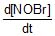= 1.6 × 10-4 M/s

Problem 2 :

The rate constant for a given reaction is k = 3 × 10-5 s-1 atm-1. Express it in units of L mol-1 sec-1.

Sol. PV = nRT ⇒ P = cRT (c : concentration in mol/L)

Substitute R = 0.0821 L-atm/mol/K ; T = 273 K ; P = 1 atm ⇒ c = 0.04462 mol/L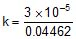= 6.73 × 10-4 L/mol/s.

Problem 3 :

From the rate laws for the reactions given below, determine the order with respect to each species and the overall order :

(i) 2HCrO4- + 6I- + 14H  → 2Cr+ 3I2+8H2O, Rate = k[HCrO4-] [I-]2 [H ]2

(ii) H2O2 +2I- +2H  → I2 +2H2O, Rate = k[H2O2] [I-]

Sol. (i) The order of the reaction with respect to [HCrO4-] is 1; with respect to [I-] is 2 and with respect to [H ] is 2. The overall order of the reaction is 1 +2 +2 = 5

(ii) The order of the reaction with respect to [H2O2] is 1 and with respect to [I-] is 1. The overall order of the reaction is 1 +1= 2.

•  In (i) stoichiometric coefficient of I- is 6 whereas the power coefficient (n) in the rate law is 2.
• Reaction (i) may not take place in a single step. It may not be possible for all the 22 molecules to be in a state to collide with each other simultaneously. Such a reaction is called a complex reaction.
•  A complex reaction takes place in a series of a number of elementary reactions.
The document Order and Molecularity of a Reaction Class 12 Notes | EduRev is a part of the Class 12 Course Chemistry Class 12.
All you need of Class 12 at this link: Class 12Use Code STAYHOME200 and get INR 200 additional OFF Use Coupon Code
All Tests, Videos & Notes of Class 12: Class 12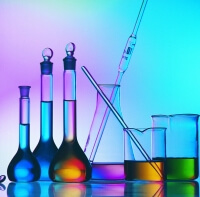## Chemistry Class 12

105 videos|264 docs|227 tests

Track your progress, build streaks, highlight & save important lessons and more!

,

,

,

,

,

,

,

,

,

,

,

,

,

,

,

,

,

,

,

,

,

;